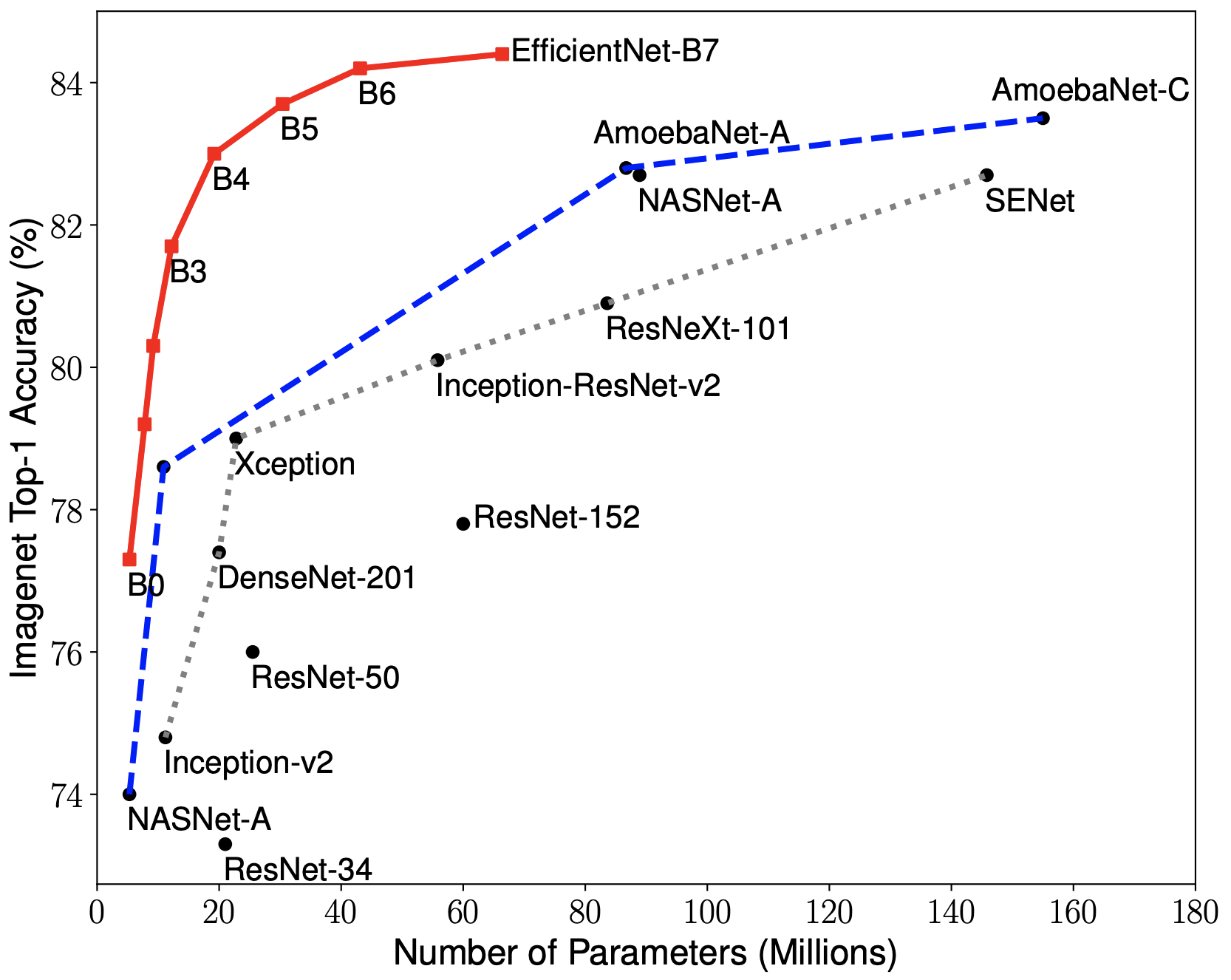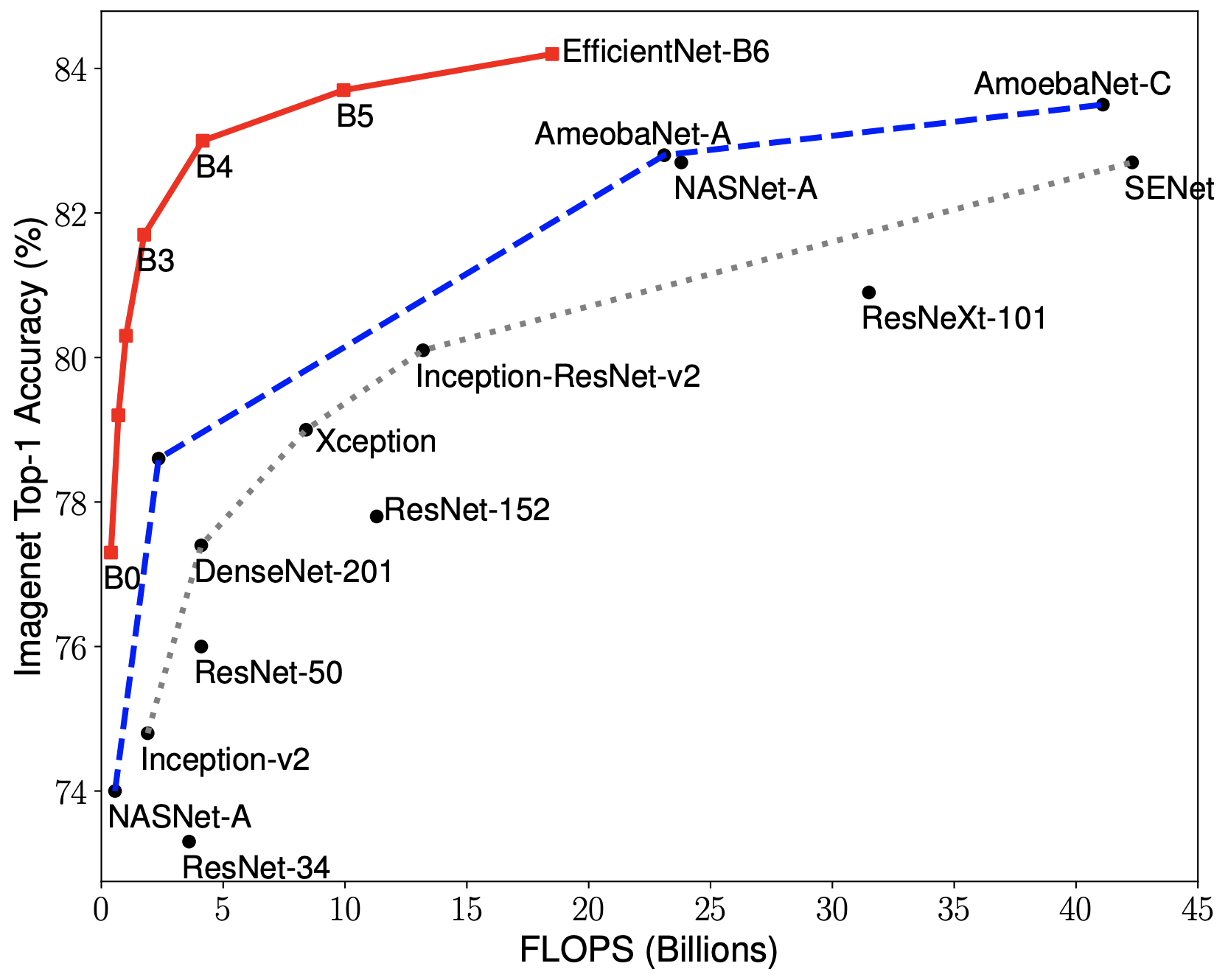Showing:

0

188

2yrs ago

5

5

MIT

# EfficientNets in KerasKeras implementation of EfficientNets from the paper EfficientNet: Rethinking Model Scaling for Convolutional Neural Networks.

Contains code to build the EfficientNets B0-B7 from the paper, and includes weights for configurations B0-B5. B6 and B7 weights will be ported when made available from the Tensorflow repository.

Supports building any other configuration model of efficient nets as well, other than the B0-B7 variants.

# Efficient Nets and Compound Coefficeint Scaling

The core idea about Efficient Nets is the use of compound scaling - using a weighted scale of three inter-connected hyper parameters of the model - Resolution of the input, Depth of the Network and Width of the Network.

When phi, the compound coefficient, is initially set to 1, we get the base configuration - in this case EfficientNetB0. We then use this configuration in a grid search to find the coefficients alpha, beta and gamma which optimize the following objective under the constraint:

Once these coefficients for alpha, beta and gamma are found, then simply scale phi, the compound coeffieints by different amounts to get a family of models with more capacity and possibly better performance.

In doing so, and using Neural Architecture Search to get the base configuration as well as great coefficients for the above, the paper generates EfficientNets, which outperform much larger and much deeper models while using less resources during both training and evaluation.# Installation

## From PyPI:

\$ pip install keras_efficientnets

## From Master branch:

pip install git+https://github.com/titu1994/keras-efficientnets.git

OR

git clone https://github.com/titu1994/keras-efficientnets.git
cd keras-efficientnets
pip install .


# Usage

Simply import keras_efficientnets and call either the model builder EfficientNet or the pre-built versions EfficientNetBX where X ranger from 0 to 7.

from keras_efficientnets import EfficientNetB0

model = EfficientNetB0(input_size, classes=1000, include_top=True, weights='imagenet')


To construct custom EfficientNets, use the EfficientNet builder. The EfficientNet builder code requires a list of BlockArgs as input to define the structure of each block in model. A default set of BlockArgs are provided in keras_efficientnets.config.

from keras_efficientnets import EfficientNet, BlockArgs

block_args_list = [
# First number is input_channels, second is output_channels.
BlockArgs(32, 16, kernel_size=3, strides=(1, 1), num_repeat=1, se_ratio=0.25, expand_ratio=1),
BlockArgs(16, 24, kernel_size=3, strides=(2, 2), num_repeat=2, se_ratio=0.25, expand_ratio=6),
...
]

model = EfficientNet(input_shape, block_args_list, ...)


# Computing Valid Compound Coefficients

In the paper, compound coefficients are obtained via simple grid search to find optimal values of alpha, beta and gamma while keeping phi as 1.

This library provides a utility function to compute valid candidates that satisfy a user defined criterion function (the one from the paper is provided as the default cost function), and quickly computes the set of hyper parameters that closely satisfy the cost function (here, MSE between the value and max cost permissible).

An example is shown below which uses the default parameters from the paper. The user can change the number of coefficients as well as the cost function itself in order to get different values of the compound coefficients.

from keras_efficientnets.optimize import optimize_coefficients
from keras_efficientnets.optimize import get_compound_coeff_func

results = optimize_coefficients(phi=1., max_cost=2.0, search_per_coeff=10)
cost_func = get_compound_coeff_func(phi=1.0, max_cost=2.0)

print("Num unique configs = ", len(results))
for i in range(10):  # print just the first 10 results out of 1000 results
print(i + 1, results[i], "Cost :", cost_func(results[i]))


Increase the number of search scopes using search_per_coeff to some larger int value. You could also combine this with tol to compute a vast set of coefficients, and then select only those that have a cost value lower than the specified tolerance.

from keras_efficientnets.optimize import optimize_coefficients
from keras_efficientnets.optimize import get_compound_coeff_func

results = optimize_coefficients(phi=1., max_cost=2.0, search_per_coeff=10, tol=1e-10)
cost_func = get_compound_coeff_func(phi=1.0, max_cost=2.0)

print("Num unique configs = ", len(results))
for i in range(10):  # print just the first 10 results out of 125 results
print(i + 1, results[i], "Cost :", cost_func(results[i]))


# Requirements

• Tensorflow 1.13+ (CPU or GPU version must be installed before installation of this library)
• Keras 2.2.4+
 Mingxing Tan and Quoc V. Le. EfficientNet: Rethinking Model Scaling for Convolutional Neural Networks. ICML 2019. Arxiv link: https://arxiv.org/abs/1905.11946.


## Rate & Review

Great Documentation0
Easy to Use0
Performant0
Highly Customizable0
Bleeding Edge0
Responsive Maintainers0
Poor Documentation0
Hard to Use0
Slow0
Buggy0
Abandoned0
Unwelcoming Community0
100